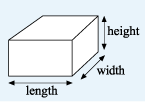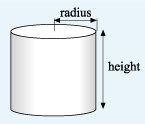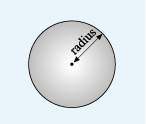Succeed with maths – Part 2

Start this free course now. Just create an account and sign in. Enrol and complete the course for a free statement of participation or digital badge if available.

Free course

# 4.1 Volume formulas

You may have already worked out that the formula for the volume of a cuboid can be calculated using the formula shown below.Figure 29 A cuboid with the length, width and height marked

Here are the formulas of various other shapes that you may encounter as well. Remember to make a note of them somewhere, as you may need one or another for the quizzes!

## Volume of cylinderFigure 30 A cylinder with the radius of the base and the height marked

## Volume of a sphereFigure 31 A sphere with the radius marked

Use the relevant formulas to complete this next activity and apply what you have learned in this section.

## Activity 8 A fuel tanker

Timing: Allow approximately 5 minutes
• a.The container on a fuel tanker is approximately cylindrical, with an internal diameter of about 2.25 m and an internal length of 10 m. Roughly how many cubic metres of fuel will it hold?

• a.

The length is 10 m, which is in this case equivalent to the height.

Substituting these values into the formula for the volume of a cylinder:

Rounding to the nearest cubic metre, the volume is 40 m3.

• b.The petrol station has an underground tank that measures 2.5 m by 4 m by 3 m. If the tank is empty, will it hold all the fuel in the tanker?

• b.The tank must be a cuboid, as there are 3 dimensions given.

Since the fuel tanker will hold about 40 m3 the petrol station’s tank is not large enough to hold all the fuel.

This activity completes your study of shapes this week.